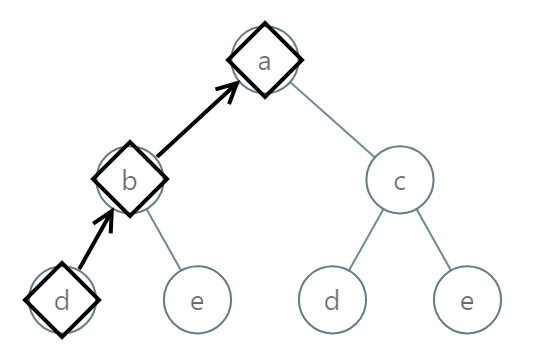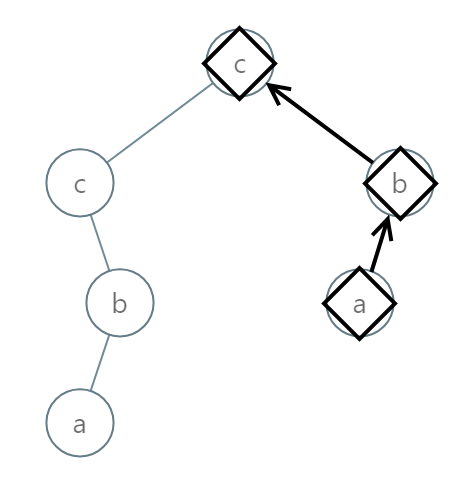# 988. Smallest String Starting From Leaf

Meidum
You are given the `root` of a binary tree where each node has a value in the range `[0, 25]` representing the letters `'a'` to `'z'`.
Return the lexicographically smallest string that starts at a leaf of this tree and ends at the root.
As a reminder, any shorter prefix of a string is lexicographically smaller.
• For example, `"ab"` is lexicographically smaller than `"aba"`.
A leaf of a node is a node that has no children.
Example 1:Input: root = [0,1,2,3,4,3,4]
Output:
"dba"
Example 2:Input: root = [25,1,3,1,3,0,2]
Output:
Example 3:Input: root = [2,2,1,null,1,0,null,0]
Output:
"abc"
Constraints:
• The number of nodes in the tree is in the range `[1, 8500]`.
• `0 <= Node.val <= 25`

### 解題

Runtime: 3 ms, faster than 100%
Memory Usage: 4.5 MB, less than 92%
/**
* Definition for a binary tree node.
* type TreeNode struct {
* Val int
* Left *TreeNode
* Right *TreeNode
* }
*/
func smallestFromLeaf(root *TreeNode) string {
return helper(root, "")
}
func helper(root *TreeNode, str string) string {
if root == nil {
return str
}
newStr := string(byte(root.Val + 97)) + str
if root.Left == nil && root.Right == nil {
return newStr
}
if root.Left == nil {
return helper(root.Right, newStr)
}
if root.Right == nil {
return helper(root.Left, newStr)
}
return min(helper(root.Left, newStr), helper(root.Right, newStr))
}
func min(a, b string) string{
if a < b {
return a
}
return b
}